# MP Board Class 10th Maths Solutions Chapter 8 Introduction to Trigonometry Ex 8.2

In this article, we will share MP Board Class 10th Maths Book Solutions Chapter 8 Introduction to Trigonometry Ex 8.2 Pdf, These solutions are solved subject experts from the latest edition books.

## MP Board Class 10th Maths Solutions Chapter 8 Introduction to Trigonometry Ex 8.2

Question 1.
Evaluate the following:
(i) sin60° cos30° + sin 30° cos 60°
(ii) 2tan2 45° + cos230° – sin260°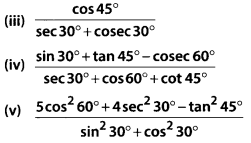Solution:
(i) We have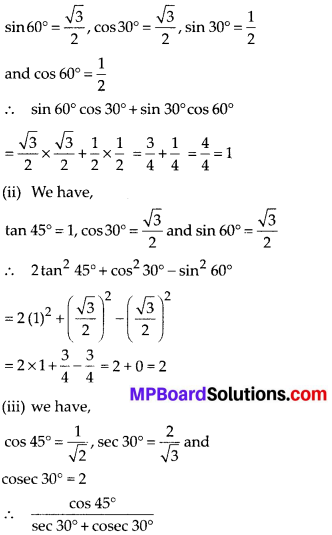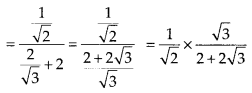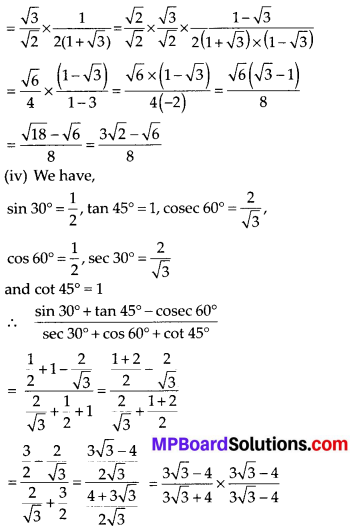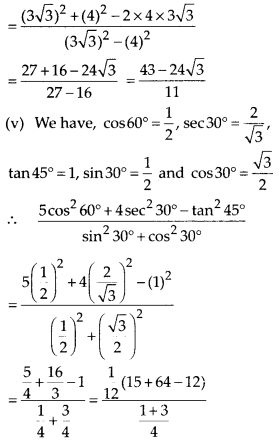= $$\frac{\frac{1}{12}\times67}{\frac{4}{4}}=\frac{67}{12}$$

Question 2.
Choose the correct option and justify your choice:
(i) $$\frac{2 \tan 30^{\circ}}{1+\tan ^{2} 30^{\circ}}=$$
(A) sin 60°
(B) cos 60°
(C) tan 60°
(D) sin 30°

(ii) $$\frac{1-\tan ^{2} 45^{\circ}}{1+\tan ^{2} 45^{\circ}}=$$
(A) tan 90°
(B) 1
(C) sin 45°
(D) 0

(iii) sin 2A = 2sin A is true when A =
(A) 0°
(B) 30°
(C) 45°
(D) 60°

(iv) $$\frac{2 \tan 30^{\circ}}{1-\tan ^{2} 30^{\circ}}=$$
(A) cos 60°
(B) sin 60°
(C) tan 60°
(D) sin 30°
Solution: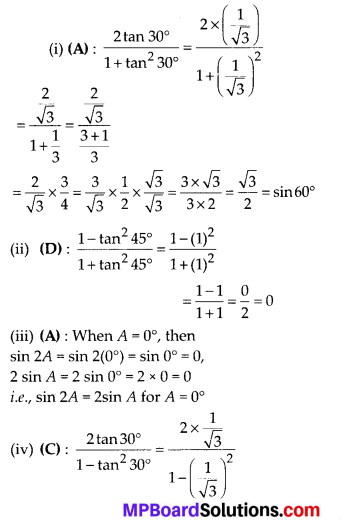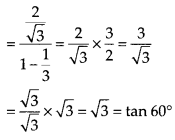Question 3.
If tan (A + B) = $$\sqrt{3}$$ and tan (A – B) = $$\frac{1}{\sqrt{3}}$$ 0°< A + B ≤ 90°; A > B, find A and B.
Solution:
We have,
tan 60° = $$\sqrt{3}$$, tan 30° = $$\frac{1}{\sqrt{3}}$$ ……………. (1)
Also, tan(A + B) = $$\sqrt{3}$$ and tan (A – B) = $$\frac{1}{\sqrt{3}}$$ …………… (2)
From (1) and (2), we get
A + B = 60° …………… (3)
and A – B = 30° ………….. (4)
On adding (3) and (4), we get
2A = 90° ⇒ A =45°
On subtracting (4) from (3), we get
2B = 30° ⇒ B = 15°

Question 4.
(i) sin (A + B) = sin A + sin B.
(ii) The value of sin6 increases as θ increases.
(iii) The value of cosθ increases as θ increases.
(iv) sin θ = cos θ for all values of θ.
(v) cot A is not defined for A = 0°.
Solution:
(i) False:
Let us take A = 30° and B = 60°, then
L.H.S = sin (30° + 60°) = sin 90° = 1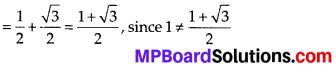∴ L.H.S. ≠ R.H.S.
(ii) True:
Since, the values of sin θ increases from 0 to 1 as θ increases from 0° to 90°.
(iii) False:
Since, the value of cos θ decreases from 1 to 0 as θ increases from 0° to 90°.
(iv) False:
Let us take θ = 30°
sin 30° = $$\frac{1}{2}$$ and cos 30° = $$\frac{\sqrt{3}}{2}$$
⇒ sin 30° ≠ cos 30°
(v) True:
We have, cot 0° = not defined